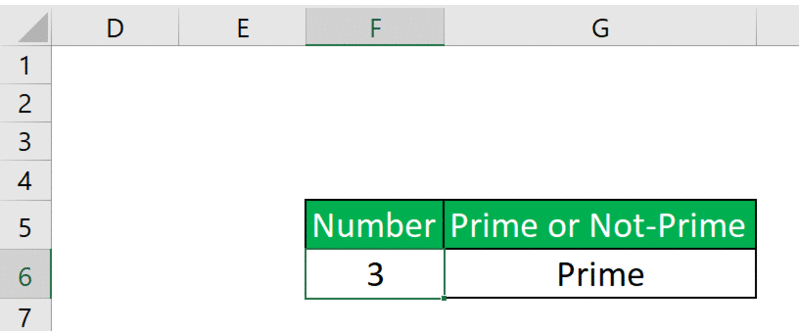Open In App

# How to Check if the Number is Prime Number in Excel?

A prime number is a number that is greater than 1 and has no positive divisor other than 1 and the number itself.

### Approach:

To check whether the number is prime or not we have to divide the number with all the numbers between 2 and the square root of that number. And if it gives remainder 0 in any of the divisions it means that the number is a positive factor and ultimately results in a non-prime number. So if any of the divisions don’t result in the remainder 0 then it means that the number doesn’t have any positive factor and ultimately results in the Prime number.

• Take the number
• If the number is 2 then it is a Prime number else follow the next steps
• Derive the square root of the number
• Take the upper bound of the square root obtained and then minus it by one
• Divide the number with all the numbers between 2 and the square root of the number
• If the remainder is 0 in any of the divisions then non-prime.
• Else it is the prime number

Formula:

`=IF(F6=2,"Prime",IF(AND(MOD(F6,ROW(OFFSET(\$C\$2,,,ROUNDUP(SQRT(F6),0)-1)))<>0),"Prime","Not Prime"))`

Let’s see each of these functions one by one so that it is clearly understood.

Step1: First of all check if the given number is 2 or not and if it is TRUE then print out that it is PRIME.

Step 2: If the number is not 2 then derive the square root of the given number.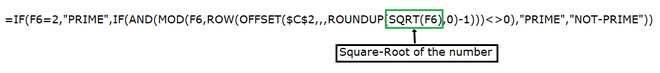Step 3: Round off the output of the square root and minus one from it ( we have to check from 2 to square root of a given number which is not included)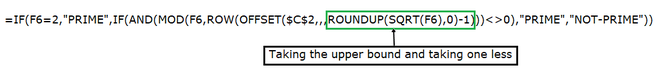Step 4: Taking the range of numbers from the 2 to the one less than the square root of the given number.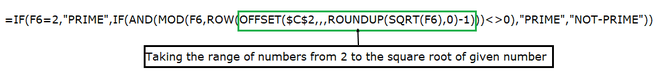Step 5: Taking one by one, the numbers from that range to evaluate further for the division.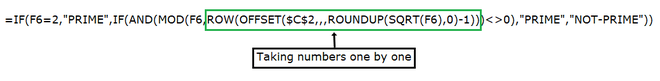Step-6: Dividing the given number from the number in the range of 2 to one less then square root of the given number and checking whether it is 0 or not. If it is 0 then the given number is NOT-PRIME and if it is not 0 then the given number is PRIME.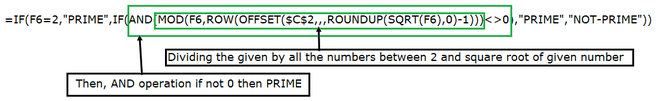Output: